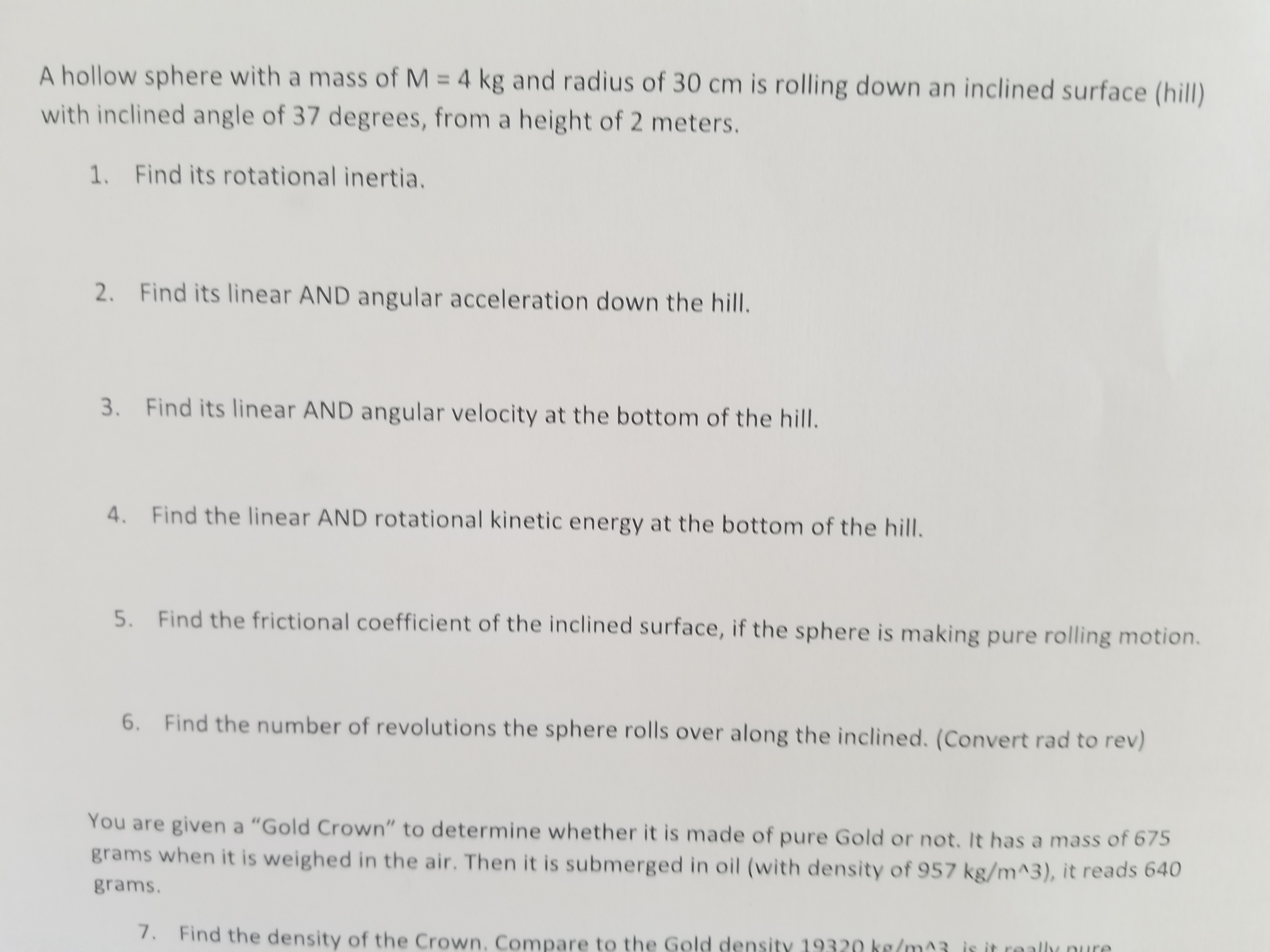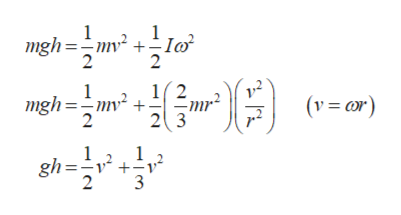# A hollow sphere with a mass of M 4 kg and radius of 30 cm is rolling down an inclined surface (hill)with inclined angle of 37 degrees, from a height of 2 meters.1. Find its rotational inertia.2.Find its linear AND angular acceleration down the hill.3.Find its linear AND angular velocity at the bottom of the hill.4. Find the linear AND rotational kinetic energy at the bottom of the hill.5.Find the frictional coefficient of the inclined surface, if the sphere is making pure rolling motion.6.Find the number of revolutions the sphere rolls over along the inclined. (Convert rad to rev)You are given a "Gold Crown" to determine whether it is made of pure Gold or not. It has a mass of 675grams when it is weighed in the air. Then it is submerged in oil (with density of 957 kg/m^3), it reads 640grams.7. Find the density of the Crown. Compare to the Gold

Question
8 views

4 5 and 6help_outlineImage TranscriptioncloseA hollow sphere with a mass of M 4 kg and radius of 30 cm is rolling down an inclined surface (hill) with inclined angle of 37 degrees, from a height of 2 meters. 1. Find its rotational inertia. 2. Find its linear AND angular acceleration down the hill. 3. Find its linear AND angular velocity at the bottom of the hill. 4. Find the linear AND rotational kinetic energy at the bottom of the hill. 5. Find the frictional coefficient of the inclined surface, if the sphere is making pure rolling motion. 6. Find the number of revolutions the sphere rolls over along the inclined. (Convert rad to rev) You are given a "Gold Crown" to determine whether it is made of pure Gold or not. It has a mass of 675 grams when it is weighed in the air. Then it is submerged in oil (with density of 957 kg/m^3), it reads 640 grams. 7. Find the density of the Crown. Compare to the Gold fullscreen
check_circle

Step 1

(4)

According to the work energy theo...help_outlineImage Transcriptionclosemgh=2o Myé + 12 1 (vr) mgh=m + 23 2 1 1 gh= +v 2 3 fullscreen

### Want to see the full answer?

See Solution

#### Want to see this answer and more?

Solutions are written by subject experts who are available 24/7. Questions are typically answered within 1 hour.*

See Solution
*Response times may vary by subject and question.
Tagged in

### Physics